stepwise regression

•5星
101KB qq_40957277 2021-09-07 22:51:15
•5星
105KB weixin_42696333 2021-09-10 17:50:39
•5星
15KB qq_33657870 2021-06-05 14:32:11
•5星
11.46MB qq_43380329 2021-01-21 21:06:58
•5星
11.8MB guoruibin123 2021-05-21 15:45:32
•5星
157KB qq_41934573 2021-05-21 23:17:41
•5星
459KB mooncrystal123 2021-01-27 17:45:03
• 230KB weixin_38643127 2021-05-24 15:30:49
• 90KB weixin_38567956 2020-09-17 20:39:24
• stepwise逐步回归 逐步回归

10KB qq_41714527 2018-02-06 11:46:52
• matlab逐步回归 逐步回归

10KB sheeryii123 2018-01-22 22:16:11
• 24.31MB qq_42167992 2020-05-21 18:05:02
• 41KB weixin_38750829 2021-05-21 12:18:26
• 用Excel作逐步回归分析_王飞凤 逐步回归

1.42MB qq_38325514 2017-10-30 21:06:39
• 104KB weixin_38675797 2021-05-21 12:06:06
• 逐步回归程序代码 逐步回归代码

47KB maxiaotiao0417 2015-09-06 21:41:20
• 99KB weixin_38665814 2021-05-21 12:21:24
• 714.63MB yanyipqng1970 2020-07-22 15:39:50
• 239B Will_Zhan 2020-01-30 16:06:25
• 918KB u013883025 2021-06-22 20:11:34
• 6KB weixin_38683930 2021-05-21 11:42:24
• 1.07MB weixin_38735570 2020-06-10 10:50:46
• 691.56MB yanyipqng1970 2020-07-22 15:54:41
• 274KB weixin_38722607 2020-02-25 05:36:29
• 7KB qq_39010714 2017-11-21 21:24:54
• 数学建模逐步回归PPT学习教案.pptx 学习课件

123KB qq_37174420 2021-10-01 08:38:02
• 多元逐步回归分析程序 回归分析

20KB u011545706 2014-09-05 20:08:42
• 逐步回归基本思想是将变量逐个引入模型，每引入一个解释变量后都要进行F检验，并对已经选入的解释变量逐个进行t检验，当原来引入的解释变量由于后面解释变量的引入变得不再显著时，则将其删除。以确保每次引入新的...

逐步回归基本思想是将变量逐个引入模型，每引入一个解释变量后都要进行F检验，并对已经选入的解释变量逐个进行t检验，当原来引入的解释变量由于后面解释变量的引入变得不再显著时，则将其删除。以确保每次引入新的变量之前回归方程中只包含显著性变量。这是一个反复的过程，直到既没有显著的解释变量选入回归方程，也没有不显著的解释变量从回归方程中剔除为止。以保证最后所得到的解释变量集是最优的。

本例的逐步回归则有所变化，没有对已经引入的变量进行t检验，只判断变量是否引入和变量是否剔除，“双重检验”逐步回归，简称逐步回归。

先来看一下逐步回归的计算步骤：1. 计算第零步增广矩阵。第零步增广矩阵是由预测因子和预测对象两两之间的相关系数构成的。

引进因子。在增广矩阵的基础上，计算每个因子的方差贡献，挑选出没有进入方程的因子中方差2. 贡献最大者对应的因子，计算该因子的方差比，查F分布表确定该因子是否引入方程。

3. 剔除因子。计算此时方程中已经引入的因子的方差贡献，挑选出方差贡献最小的因子,计算该因子的方差比，查F分布表确定该因子是否从方程中剔除。

4. 矩阵变换。将第零步矩阵按照引入方程的因子序号进行矩阵变换，变换后的矩阵再次进行引进因子和剔除因子的步骤，直到无因子可以引进，也无因子可以剔除为止，终止逐步回归分析计算。

a.以下代码实现了数据的读取，相关系数的计算子程序和生成第零步增广矩阵的子程序

注意：pandas库读取csv的数据结构为DataFrame结构，此处转化为numpy中的(n-dimension array，ndarray)数组进行计算

import numpy as np

import pandas as pd

#数据读取

#利用pandas读取csv，读取的数据为DataFrame对象

# 将DataFrame对象转化为数组,数组的最后一列为预报对象

data= data.values.copy()

# print(data)

# 计算回归系数，参数

def get_regre_coef(X,Y):

S_xy=0

S_xx=0

S_yy=0

# 计算预报因子和预报对象的均值

X_mean = np.mean(X)

Y_mean = np.mean(Y)

for i in range(len(X)):

S_xy += (X[i] - X_mean) * (Y[i] - Y_mean)

S_xx += pow(X[i] - X_mean, 2)

S_yy += pow(Y[i] - Y_mean, 2)

return S_xy/pow(S_xx*S_yy,0.5)

#构建原始增广矩阵

def get_original_matrix():

# 创建一个数组存储相关系数,data.shape几行(维)几列，结果用一个tuple表示

# print(data.shape)

col=data.shape

# print(col)

r=np.ones((col,col))#np.ones参数为一个元组(tuple)

# print(np.ones((col,col)))

# for row in data.T:#运用数组的迭代，只能迭代行，迭代转置后的数组，结果再进行转置就相当于迭代了每一列

# print(row.T)

for i in range(col):

for j in range(col):

r[i,j]=get_regre_coef(data[:,i],data[:,j])

return r

b.第二部分主要是计算公差贡献和方差比

def get_vari_contri(r):

col = data.shape

#创建一个矩阵来存储方差贡献值

v=np.ones((1,col-1))

# print(v)

for i in range(col-1):

# v[0,i]=pow(r[i,col-1],2)/r[i,i]

v[0, i] = pow(r[i, col - 1], 2) / r[i, i]

return v

#选择因子是否进入方程，

#参数说明：r为增广矩阵，v为方差贡献值，k为方差贡献值最大的因子下标,p为当前进入方程的因子数

def select_factor(r,v,k,p):

row=data.shape#样本容量

col=data.shape-1#预报因子数

#计算方差比

f=(row-p-2)*v[0,k-1]/(r[col,col]-v[0,k-1])

# print(calc_vari_contri(r))

return f

c.第三部分调用定义的函数计算方差贡献值

#计算第零步增广矩阵

r=get_original_matrix()

# print(r)

#计算方差贡献值

v=get_vari_contri(r)

print(v)

#计算方差比

计算结果：此处没有编写判断方差贡献最大的子程序，因为在其他计算中我还需要变量的具体物理含义所以不能单纯的由计算决定对变量的取舍，此处看出第四个变量的方查贡献最大

# #计算方差比

# print(data.shape)

f=select_factor(r,v,4,0)

print(f)

#######输出##########

22.79852020138227

计算第四个预测因子的方差比(粘贴在了代码中)，并查F分布表3.280进行比对,22.8>3.28,引入第四个预报因子。(前三次不进行剔除椅子的计算)

d.第四部分进行矩阵的变换

#逐步回归分析与计算

#通过矩阵转换公式来计算各部分增广矩阵的元素值

def convert_matrix(r,k):

col=data.shape

k=k-1#从第零行开始计数

#第k行的元素单不属于k列的元素

r1 = np.ones((col, col)) # np.ones参数为一个元组(tuple)

for i in range(col):

for j in range(col):

if (i==k and j!=k):

r1[i,j]=r[k,j]/r[k,k]

elif (i!=k and j!=k):

r1[i,j]=r[i,j]-r[i,k]*r[k,j]/r[k,k]

elif (i!= k and j== k):

r1[i,j] = -r[i,k]/r[k,k]

else:

r1[i,j] = 1/r[k,k]

return r1

e.进行完矩阵变换就循环上面步骤进行因子的引入和剔除

再次计算各因子的方差贡献前三个未引入方程的方差因子进行排序，得到第一个因子的方差贡献最大，计算第一个预报因子的F检验值，大于临界值引入第一个预报因子进入方程。

#矩阵转换，计算第一步矩阵

r=convert_matrix(r,4)

# print(r)

#计算第一步方差贡献值

v=get_vari_contri(r)

#print(v)

f=select_factor(r,v,1,1)

print(f)

#########输出#####

108.22390933074443

进行矩阵变换，计算方差贡献可以看出还没有引入方程的因子2和3，方差贡献较大的是因子2，计算因子2的f检验值5.026>3.28,故引入预报因子2

f=select_factor(r,v,2,2)

print(f)

##########输出#########

5.025864648951804

继续进行矩阵转换，计算方差贡献这一步需要考虑剔除因子了，有方差贡献可以知道，已引入方程的因子中方差贡献最小的是因子4，分别计算因子3的引进f检验值0.0183

和因子4的剔除f检验值1.863，均小于3.28(查F分布表)因子3不能引入，因子4需要剔除，此时方程中引入的因子数为2

#选择是否剔除因子，

#参数说明：r为增广矩阵，v为方差贡献值，k为方差贡献值最大的因子下标,t为当前进入方程的因子数

def delete_factor(r,v,k,t):

row = data.shape # 样本容量

col = data.shape - 1 # 预报因子数

# 计算方差比

f = (row - t - 1) * v[0, k - 1] / r[col, col]

# print(calc_vari_contri(r))

return f

#因子3的引进检验值0.018233473487350636

f=select_factor(r,v,3,3)

print(f)

#因子4的剔除检验值1.863262422188088

f=delete_factor(r,v,4,3)

print(f)

在此对矩阵进行变换，计算方差贡献，已引入因子(因子1和2)方差贡献最小的是因子1，为引入因子方差贡献最大的是因子4，计算这两者的引进f检验值和剔除f检验值

#因子4的引进检验值1.8632624221880876，小于3.28不能引进

f=select_factor(r,v,4,2)

print(f)

#因子1的剔除检验值146.52265486251397,大于3.28不能剔除

f=delete_factor(r,v,1,2)

print(f)

不能剔除也不能引进变量，此时停止逐步回归的计算。引进方程的因子为预报因子1和预报因子2，借助上一篇博客写的多元回归。对进入方程的预报因子和预报对象进行多元回归。输出多元回归的预测结果，一次为常数项，第一个因子的预测系数，第二个因子的预测系数。

#因子1和因子2进入方程

#对进入方程的预报因子进行多元回归

# regs=LinearRegression()

X=data[:,0:2]

Y=data[:,4]

X=np.mat(np.c_[np.ones(X.shape),X])#为系数矩阵增加常数项系数

Y=np.mat(Y)#数组转化为矩阵

#print(X)

B=np.linalg.inv(X.T*X)*(X.T)*(Y.T)

print(B.T)#输出系数,第一项为常数项，其他为回归系数

###输出##

#[[52.57734888 1.46830574 0.66225049]]

对于初学者想更轻松的学好Python开发，爬虫技术，Python数据分析，人工智能等技术，我准备了一套系统教学资源，希望对你们有帮助~2021最新版Python零基础高强度7天极速入门到精通_哔哩哔哩 (゜-゜)つロ 干杯~-bilibili​www.bilibili.com2021最新版python爬虫7天速成(附带代码课件)_哔哩哔哩 (゜-゜)つロ 干杯~-bilibili​www.bilibili.com清华大学博士2020最新Python数据结构与算法教程(20小时全套合集)_哔哩哔哩 (゜-゜)つロ 干杯~-bilibili​www.bilibili.com展开全文weixin_32955455 2021-01-13 09:28:23
• 34KB weixin_38576922 2021-05-21 12:17:48
• 39KB weixin_38639471 2021-05-21 12:18:21
• 在这种情况下可用逐步回归分析，进行x因子的筛选，这样建立的多元回归模型预测效果会更较好。逐步回归分析，首先要建立因变量y与自变量x之间的总回归方程，再对总的方程及每—个自变量进行假设检验。当总的方程不...

在自变量很多时，其中有的因素可能对应变量的影响不是很大，而且x之间可能不完全相互独立的，可能有种种互作关系。在这种情况下可用逐步回归分析，进行x因子的筛选，这样建立的多元回归模型预测效果会更较好。

逐步回归分析，首先要建立因变量y与自变量x之间的总回归方程，再对总的方程及每—个自变量进行假设检验。当总的方程不显著时，表明该多元回归方程线性关系不成立；而当某—个自变量对y影响不显著时，应该把它剔除，重新建立不包含该因子的多元回归方程。筛选出有显著影响的因子作为自变量，并建立“最优”回归方程。

回归方程包含的自变量越多，回归平方和越大，剩余的平方和越小，剩余均方也随之较小，预测值的误差也愈小，模拟的效果愈好。但是方程中的变量过多，预报工作量就会越大，其中有些相关性不显著的预报因子会影响预测的效果。因此在多元回归模型中，选择适宜的变量数目尤为重要。

逐步回归在病虫预报中的应用实例:

以陕西省长武地区1984~1995年的烟蚜传毒病情资料、相关虫情和气象资料为例(数据见DATA6.xls)，建立蚜传病毒病情指数的逐步回归模型，说明逐步回归分析的具体步骤。影响蚜传病毒病情指数的虫情因子和气象因子一共有21个，通过逐步回归，从中选出对病情指数影响显著的因子，从而建立相应的模型。对1984~1995年的病情指数进行回检，然后对1996~1998年的病情进行预报，再检验预报的效果。

变量说明如下：

y：历年病情指数

x1：前年冬季油菜越冬时的蚜量(头/株)

x2：前年冬季极端气温

x3：5月份最高气温

x4：5月份最低气温

x5：3~5月份降水量

x6：4~6月份降水量

x7：3~5月份均温

x8：4~6月份均温

x9：4月份降水量

x10：4月份均温

x11：5月份均温

x12：5月份降水量

x13：6月份均温

x14：6月份降水量

x15：第一次蚜迁高峰期百株烟草有翅蚜量

x16：5月份油菜百株蚜量

x17：7月份降水量

x18：8月份降水量

x19：7月份均温

x20：8月份均温

x21：元月均温

1)准备分析数据

在SPSS数据编辑窗口中，用“File→Open→Data”命令，打开“DATA6.xls”数据文件。数据工作区如下图3-1显示。图3-1

2)启动线性回归过程

单击SPSS主菜单的“Analyze”下的“Regression”中“Linear”项，将打开如图3-2所示的线性回归过程窗口。图3-2

线性回归对话窗口

3)

设置分析变量

设置因变量：将左边变量列表中的“y”变量，选入到“Dependent”因变量显示栏里。

设置自变量：将左边变量列表中的“x1”~“x21”变量，全部选移到“Independent(S)”自变量栏里。

设置控制变量:

本例子中不使用控制变量，所以不选择任何变量。

选择标签变量:

选择“年份”为标签变量。

选择加权变量:

本例子没有加权变量，因此不作任何设置。

4)回归方式

在“Method”分析方法框中选中“Stepwise”逐步分析方法。该方法是根据“Options”选择对话框中显著性检验(F)的设置，在方程中进入或剔除单个变量，直到所建立的方程中不再含有可加入或可剔除的变量为止。设置后的对话窗口如图3-3。图3-3

5)设置变量检验水平

在图6-15主对话框里单击“Options”按钮，将打开如图3-4所示的对话框。图3-4

“Stepping

Method Criteria”框里的设置用于逐步回归分析的选择标准。

其中“Use

probability of

F”选项，提供设置显著性F检验的概率。如果一个变量的F检验概率小于或等于进入“Entry”栏里设置的值，那么这个变量将被选入回归方程中；当回归方程中变量的F值检验概率大于剔除“Removal”栏里设置的值，则该变量将从回归方程中被剔除。由此可见，设置F检验概率时，应使进入值小于剔除值。

“Ues F

value” 选项，提供设置显著性F检验的分布值。如果一个变量的F值大于所设置的进入值(Entry)，那么这个变量将被选入回归方程中；当回归方程中变量的F值小于设置的剔除值(Removal)，则该变量将从回归方程中被剔除。同时，设置F分布值时，应该使进入值大于剔除值。

本例子使用显著性F检验的概率，在进入“Entry”栏里设置为“0.15”，在剔除“Removal”栏里设置为“0.20”(剔除的概率值应比进入的值大)，如图6-17所示。

图6-17窗口中的其它设置参照一元回归设置。

6)设置输出统计量

在主对话图3-2窗口中，单击“Statistics”按钮，将打开如图6-18所示的对话框。该对话框用于设置相关参数。其中各项的意义分别为：图3-5

“Statistics”对话框

①“Regression

Coefficients”回归系数选项：“Estimates”输出回归系数和相关统计量。“Confidence

interval”回归系数的95%置信区间。“Covariance

matrix”回归系数的方差-协方差矩阵。

本例子选择“Estimates”输出回归系数和相关统计量。

②“Residuals”残差选项：“Durbin-Watson”Durbin-Watson检验。“Casewise

diagnostic”输出满足选择条件的观测量的相关信息。选择该项，下面两项处于可选状态：“Outliers

outside standard deviations”选择标准化残差的绝对值大于输入值的观测量；“All

cases”选择所有观测量。

本例子都不选。

③ 其它输入选项“Model

fit”输出相关系数、相关系数平方、调整系数、估计标准误、ANOVA表。“R

squared change”输出由于加入和剔除变量而引起的复相关系数平方的变化。“Descriptives”输出变量矩阵、标准差和相关系数单侧显著性水平矩阵。“Part

and partial correlation”相关系数和偏相关系数。“Collinearity

diagnostics”显示单个变量和共线性分析的公差。

本例子选择“Model

fit”项。

7)绘图选项

在主对话框单击“Plots”按钮，将打开如图3-6所示的对话框窗口。该对话框用于设置要绘制的图形的参数。图中的“X”和“Y”框用于选择X轴和Y轴相应的变量。图3-6“Plots”绘图对话框窗口

左上框中各项的意义分别为：

“DEPENDNT”因变量。

“ZPRED”标准化预测值。

“ZRESID”标准化残差。

“DRESID”删除残差。

“SRESID”学生氏化残差。

“SDRESID”学生氏化删除残差。

“Standardized

Residual Plots”设置各变量的标准化残差图形输出。其中共包含两个选项：“Histogram”用直方图显示标准化残差。“Normal

probability plots”比较标准化残差与正态残差的分布示意图。

“Produce

all partial plot”偏残差图。对每一个自变量生成其残差对因变量残差的散点图。

本例子不作绘图，不选择。

8)

保存分析数据的选项

在主对话框里单击“Save”按钮，将打开如图3-7所示的对话框。图3-7“Save”对话框

①“Predicted

Values”预测值栏选项：Unstandardized

非标准化预测值。就会在当前数据文件中新添加一个以字符“PRE_”开头命名的变量，存放根据回

归模型拟合的预测值。Standardized

标准化预测值。Adjusted

调整后预测值。S.E.

of mean predictions 预测值的标准误。

本例选中“Unstandardized”非标准化预测值。

②“Distances”距离栏选项：Mahalanobis:

距离。Cook’s”:

Cook距离。Leverage

values: 杠杆值。

③“Prediction

Intervals”预测区间选项：Mean:

区间的中心位置。Individual:

观测量上限和下限的预测区间。在当前数据文件中新添加一个以字符“LICI_”开头命名的变量，存放

预测区间下限值；以字符“UICI_”开头命名的变量，存放预测区间上限值。Confidence

Interval：置信度。

本例不选。

④“Save

to New File”保存为新文件：

选中“Coefficient

statistics”项将回归系数保存到指定的文件中。本例不选。

“Export model information to XML

file” 导出统计过程中的回归模型信息到指定文件。本例不选。

⑥“Residuals” 保存残差选项：“Unstandardized”非标准化残差。“Standardized”标准化残差。“Studentized”学生氏化残差。“Deleted”删除残差。“Studentized

deleted”学生氏化删除残差。

本例不选。

⑦“Influence

Statistics” 统计量的影响。“DfBeta(s)”删除一个特定的观测值所引起的回归系数的变化。“Standardized

DfBeta(s)”标准化的DfBeta值。“DiFit”

删除一个特定的观测值所引起的预测值的变化。“Standardized

DiFit”标准化的DiFit值。“Covariance

ratio”删除一个观测值后的协方差矩隈的行列式和带有全部观测值的协方差矩阵的行列式的比率。

本例子不保存任何分析变量，不选择。

9)提交执行

在主对话框里单击“OK”，提交执行，结果将显示在输出窗口中。主要结果见表6-10至表6-13。

10)

结果分析

主要结果:表6-10

是逐步回归每一步进入或剔除回归模型中的变量情况。表6-11

是逐步回归每一步的回归模型的统计量：R 是相关系数；R Square

Square 调整后的判定系数；Std. Error of the Estimate 估计标准误差。表6-12

是逐步回归每一步的回归模型的方差分析，F值为10.930，显著性概率是0.001，表明回归极显著。表6-13

是逐步回归每一步的回归方程系数表。

分析:

建立回归模型：

根据多元回归模型：从6-13中看出，过程一共运行了四步，最后一步以就是表中的第4步的计算结果得知：21个变量中只进入了4个变量x15、x4、x7 和 x5。

把表6-13中“非标准化回归系数”栏目中的“B”列数据代入多元回归模型得到预报方程：预测值的标准差可用剩余标准差估计：回归方程的显著性检验：

从表6-12方差分析表第4模型中得知：F统计量为622.72，系统自动检验的显著性水平为0.0000(非常小)。

F(0.00001,4,7)值为70.00。因此回归方程相关非常非常显著。

由回归方程式可以看出，在陕西长武烟草蚜传病毒病8月份的病情指数(y)与x4(5月份最低气温)、x15(第一次蚜迁高峰期百株烟草有翅蚜量)呈显著正相关，而与x5(3~5月份降水量)和x7 (3~5月份均温)呈显著负相关。

通过大田调查结果表明，烟草蚜传病毒病发生与蚜虫的迁飞有密切的关系。迁入烟田的有翅蚜有两次高峰期，呈双峰曲线。第一高峰期出现在5月中旬至6月初，此次迁飞的高峰期与大田发病率呈显著正相关。第二高峰期在6月上旬末至6月中旬，此次迁飞高峰期与大田发病率关系不大。5月份的最低气温(x4)和3~5月份均温(x7 )通过影响传媒介体蚜虫的活动来影响田间发病。而第一次蚜迁高峰期百株烟草有翅蚜量(x15)是影响烟草蚜传病毒病病情指数(y)的重要因子。3~5月份降水量(x5)通过影响田间蚜虫传病毒病发病植株的症状表现影响大田发病程度。cda数据分析培训

展开全文weixin_39973410 2020-12-08 14:43:28
• 55KB weixin_38703123 2021-05-21 12:19:10
• 54KB weixin_38546622 2021-05-21 12:22:50
• 多元逐步回归算法 python 多元逐步回归

先谈一下个人对多元逐步回归的理解：多元逐步回归的最本质的核心是最小二乘原理，本方法中调用smf方法。 # encoding: utf-8 """ 功能：多元逐步回归 描述：基于python实现多元逐步回归的功能 作者：CHEN_C_W ...
先谈一下个人对多元逐步回归的理解：多元逐步回归的最本质的核心是最小二乘原理，本方法中调用smf方法。

# encoding: utf-8

"""
功能：多元逐步回归
描述：基于python实现多元逐步回归的功能
作者：CHEN_C_W （草木陈）
时间：2019年4月12日(星期五) 凌晨
地点：杭州
参考：https://www.cnblogs.com/lantingg/p/9535010.html
"""
import numpy as np
import pandas as pd
from sklearn.preprocessing import StandardScaler
from statsmodels.formula import api as smf

class FeatureSelection():
def stepwise(self, df, response, intercept=True, normalize=False, criterion='bic',
f_pvalue_enter=.05, p_value_enter=.05, direction='backward', show_step=True,
criterion_enter=None, criterion_remove=None, max_iter=200, **kw):

"""
逐步回归。

参数
----
df : dataframe
分析用数据框，response为第一列。
response : str
回归分析相应变量。
intercept : bool, 默认是True
模型是否有截距项。
criterion : str, 默认是'bic'
逐步回归优化规则。
f_pvalue_enter : float, 默认是.05
当选择criterion=’ssr‘时，模型加入或移除变量的f_pvalue阈值。
p_value_enter : float, 默认是.05
当选择derection=’both‘时，移除变量的pvalue阈值。
direction : str, 默认是'backward'
逐步回归方向。
show_step : bool, 默认是True
是否显示逐步回归过程。
criterion_enter : float, 默认是None
当选择derection=’both‘或'forward'时，模型加入变量的相应的criterion阈值。
criterion_remove : float, 默认是None
当选择derection='backward'时，模型移除变量的相应的criterion阈值。
max_iter : int, 默认是200
模型最大迭代次数。
"""
criterion_list = ['bic', 'aic', 'ssr', 'rsquared', 'rsquared_adj']
if criterion not in criterion_list:
raise IOError('请输入正确的criterion, 必须是以下内容之一：', '\n', criterion_list)

direction_list = ['backward', 'forward', 'both']
if direction not in direction_list:
raise IOError('请输入正确的direction, 必须是以下内容之一：', '\n', direction_list)

# 默认p_enter参数
p_enter = {'bic': 0.0, 'aic': 0.0, 'ssr': 0.05, 'rsquared': 0.05, 'rsquared_adj': -0.05}
if criterion_enter:  # 如果函数中对p_remove相应key传参，则变更该参数
p_enter[criterion] = criterion_enter

# 默认p_remove参数
p_remove = {'bic': 0.01, 'aic': 0.01, 'ssr': 0.1, 'rsquared': 0.05, 'rsquared_adj': -0.05}
if criterion_remove:  # 如果函数中对p_remove相应key传参，则变更该参数
p_remove[criterion] = criterion_remove

if normalize:  # 如果需要标准化数据
intercept = False  # 截距强制设置为0
df_std = StandardScaler().fit_transform(df)
df = pd.DataFrame(df_std, columns=df.columns, index=df.index)

""" forward """
if direction == 'forward':
remaining = list(df.columns)  # 自变量集合
remaining.remove(response)
selected = []  # 初始化选入模型的变量列表
# 初始化当前评分,最优新评分
if intercept:  # 是否有截距
formula = "{} ~ {} + 1".format(response, remaining)
else:
formula = "{} ~ {} - 1".format(response, remaining)

result = smf.ols(formula, df).fit()  # 最小二乘法回归模型拟合
current_score = eval('result.' + criterion)
best_new_score = eval('result.' + criterion)

if show_step:
print('\nstepwise starting:\n')
iter_times = 0
# 当变量未剔除完，并且当前评分更新时进行循环
while remaining and (current_score == best_new_score) and (iter_times < max_iter):
scores_with_candidates = []  # 初始化变量以及其评分列表
for candidate in remaining:  # 在未剔除的变量中每次选择一个变量进入模型，如此循环
if intercept:  # 是否有截距
formula = "{} ~ {} + 1".format(response, ' + '.join(selected + [candidate]))
else:
formula = "{} ~ {} - 1".format(response, ' + '.join(selected + [candidate]))

result = smf.ols(formula, df).fit()  # 最小二乘法回归模型拟合
fvalue = result.fvalue
f_pvalue = result.f_pvalue
score = eval('result.' + criterion)
scores_with_candidates.append((score, candidate, fvalue, f_pvalue))  # 记录此次循环的变量、评分列表

if criterion == 'ssr':  # 这几个指标取最小值进行优化
scores_with_candidates.sort(reverse=True)  # 对评分列表进行降序排序
best_new_score, best_candidate, best_new_fvalue, best_new_f_pvalue = scores_with_candidates.pop()  # 提取最小分数及其对应变量
if ((current_score - best_new_score) > p_enter[criterion]) and (
best_new_f_pvalue < f_pvalue_enter):  # 如果当前评分大于最新评分
remaining.remove(best_candidate)  # 从剩余未评分变量中剔除最新最优分对应的变量
selected.append(best_candidate)  # 将最新最优分对应的变量放入已选变量列表
current_score = best_new_score  # 更新当前评分
if show_step:  # 是否显示逐步回归过程
print('Adding %s, SSR = %.3f, Fstat = %.3f, FpValue = %.3e' %
(best_candidate, best_new_score, best_new_fvalue, best_new_f_pvalue))
elif (current_score - best_new_score) >= 0 and (
best_new_f_pvalue < f_pvalue_enter) and iter_times == 0:  # 当评分差大于等于0，且为第一次迭代
remaining.remove(best_candidate)
selected.append(best_candidate)
current_score = best_new_score
if show_step:  # 是否显示逐步回归过程
print('Adding %s, %s = %.3f' % (best_candidate, criterion, best_new_score))
elif (best_new_f_pvalue < f_pvalue_enter) and iter_times == 0:  # 当评分差小于p_enter，且为第一次迭代
selected.append(remaining)
remaining.remove(remaining)
if show_step:  # 是否显示逐步回归过程
print('Adding %s, %s = %.3f' % (remaining, criterion, best_new_score))
elif criterion in ['bic', 'aic']:  # 这几个指标取最小值进行优化
scores_with_candidates.sort(reverse=True)  # 对评分列表进行降序排序
best_new_score, best_candidate, best_new_fvalue, best_new_f_pvalue = scores_with_candidates.pop()  # 提取最小分数及其对应变量
if (current_score - best_new_score) > p_enter[criterion]:  # 如果当前评分大于最新评分
remaining.remove(best_candidate)  # 从剩余未评分变量中剔除最新最优分对应的变量
selected.append(best_candidate)  # 将最新最优分对应的变量放入已选变量列表
current_score = best_new_score  # 更新当前评分
# print(iter_times)
if show_step:  # 是否显示逐步回归过程
print('Adding %s, %s = %.3f' % (best_candidate, criterion, best_new_score))
elif (current_score - best_new_score) >= 0 and iter_times == 0:  # 当评分差大于等于0，且为第一次迭代
remaining.remove(best_candidate)
selected.append(best_candidate)
current_score = best_new_score
if show_step:  # 是否显示逐步回归过程
print('Adding %s, %s = %.3f' % (best_candidate, criterion, best_new_score))
elif iter_times == 0:  # 当评分差小于p_enter，且为第一次迭代
selected.append(remaining)
remaining.remove(remaining)
if show_step:  # 是否显示逐步回归过程
print('Adding %s, %s = %.3f' % (remaining, criterion, best_new_score))
else:
scores_with_candidates.sort()
best_new_score, best_candidate, best_new_fvalue, best_new_f_pvalue = scores_with_candidates.pop()
if (best_new_score - current_score) > p_enter[criterion]:
remaining.remove(best_candidate)
selected.append(best_candidate)
current_score = best_new_score
print(iter_times, flush=True)
if show_step:  # 是否显示逐步回归过程
print('Adding %s, %s = %.3f' % (best_candidate, criterion, best_new_score))
elif (best_new_score - current_score) >= 0 and iter_times == 0:  # 当评分差大于等于0，且为第一次迭代
remaining.remove(best_candidate)
selected.append(best_candidate)
current_score = best_new_score
if show_step:  # 是否显示逐步回归过程
print('Adding %s, %s = %.3f' % (best_candidate, criterion, best_new_score))
elif iter_times == 0:  # 当评分差小于p_enter，且为第一次迭代
selected.append(remaining)
remaining.remove(remaining)
if show_step:  # 是否显示逐步回归过程
print('Adding %s, %s = %.3f' % (remaining, criterion, best_new_score))
iter_times += 1

if intercept:  # 是否有截距
formula = "{} ~ {} + 1".format(response, ' + '.join(selected))
else:
formula = "{} ~ {} - 1".format(response, ' + '.join(selected))

self.stepwise_model = smf.ols(formula, df).fit()  # 最优模型拟合

if show_step:  # 是否显示逐步回归过程
print('\nLinear regression model:', '\n  ', self.stepwise_model.model.formula)
print('\n', self.stepwise_model.summary())

""" backward """
if direction == 'backward':
remaining, selected = set(df.columns), set(df.columns)  # 自变量集合
remaining.remove(response)
selected.remove(response)  # 初始化选入模型的变量列表
# 初始化当前评分,最优新评分
if intercept:  # 是否有截距
formula = "{} ~ {} + 1".format(response, ' + '.join(selected))
else:
formula = "{} ~ {} - 1".format(response, ' + '.join(selected))

result = smf.ols(formula, df).fit()  # 最小二乘法回归模型拟合
current_score = eval('result.' + criterion)
worst_new_score = eval('result.' + criterion)

if show_step:
print('\nstepwise starting:\n')
iter_times = 0
# 当变量未剔除完，并且当前评分更新时进行循环
while remaining and (current_score == worst_new_score) and (iter_times < max_iter):
scores_with_eliminations = []  # 初始化变量以及其评分列表
for elimination in remaining:  # 在未剔除的变量中每次选择一个变量进入模型，如此循环
if intercept:  # 是否有截距
formula = "{} ~ {} + 1".format(response, ' + '.join(selected - set(elimination)))
else:
formula = "{} ~ {} - 1".format(response, ' + '.join(selected - set(elimination)))

result = smf.ols(formula, df).fit()  # 最小二乘法回归模型拟合
fvalue = result.fvalue
f_pvalue = result.f_pvalue
score = eval('result.' + criterion)
scores_with_eliminations.append((score, elimination, fvalue, f_pvalue))  # 记录此次循环的变量、评分列表

if criterion == 'ssr':  # 这几个指标取最小值进行优化
scores_with_eliminations.sort(reverse=False)  # 对评分列表进行降序排序
worst_new_score, worst_elimination, worst_new_fvalue, worst_new_f_pvalue = scores_with_eliminations.pop()  # 提取最小分数及其对应变量
if ((worst_new_score - current_score) < p_remove[criterion]) and (
worst_new_f_pvalue < f_pvalue_enter):  # 如果当前评分大于最新评分
remaining.remove(worst_elimination)  # 从剩余未评分变量中剔除最新最优分对应的变量
selected.remove(worst_elimination)  # 从已选变量列表中剔除最新最优分对应的变量
current_score = worst_new_score  # 更新当前评分
if show_step:  # 是否显示逐步回归过程
print('Removing %s, SSR = %.3f, Fstat = %.3f, FpValue = %.3e' %
(worst_elimination, worst_new_score, worst_new_fvalue, worst_new_f_pvalue))
elif criterion in ['bic', 'aic']:  # 这几个指标取最小值进行优化
scores_with_eliminations.sort(reverse=False)  # 对评分列表进行降序排序
worst_new_score, worst_elimination, worst_new_fvalue, worst_new_f_pvalue = scores_with_eliminations.pop()  # 提取最小分数及其对应变量
if (worst_new_score - current_score) < p_remove[criterion]:  # 如果评分变动不显著
remaining.remove(worst_elimination)  # 从剩余未评分变量中剔除最新最优分对应的变量
selected.remove(worst_elimination)  # 从已选变量列表中剔除最新最优分对应的变量
current_score = worst_new_score  # 更新当前评分
if show_step:  # 是否显示逐步回归过程
print('Removing %s, %s = %.3f' % (worst_elimination, criterion, worst_new_score))
else:
scores_with_eliminations.sort(reverse=True)
worst_new_score, worst_elimination, worst_new_fvalue, worst_new_f_pvalue = scores_with_eliminations.pop()
if (current_score - worst_new_score) < p_remove[criterion]:
remaining.remove(worst_elimination)
selected.remove(worst_elimination)
current_score = worst_new_score
if show_step:  # 是否显示逐步回归过程
print('Removing %s, %s = %.3f' % (worst_elimination, criterion, worst_new_score))
iter_times += 1

if intercept:  # 是否有截距
formula = "{} ~ {} + 1".format(response, ' + '.join(selected))
else:
formula = "{} ~ {} - 1".format(response, ' + '.join(selected))

self.stepwise_model = smf.ols(formula, df).fit()  # 最优模型拟合

if show_step:  # 是否显示逐步回归过程
print('\nLinear regression model:', '\n  ', self.stepwise_model.model.formula)
print('\n', self.stepwise_model.summary())

""" both """
if direction == 'both':
remaining = list(df.columns)  # 自变量集合
remaining.remove(response)
selected = []  # 初始化选入模型的变量列表
# 初始化当前评分,最优新评分
if intercept:  # 是否有截距
formula = "{} ~ {} + 1".format(response, remaining)
else:
formula = "{} ~ {} - 1".format(response, remaining)

result = smf.ols(formula, df).fit()  # 最小二乘法回归模型拟合
current_score = eval('result.' + criterion)
best_new_score = eval('result.' + criterion)

if show_step:
print('\nstepwise starting:\n')
# 当变量未剔除完，并且当前评分更新时进行循环
iter_times = 0
while remaining and (current_score == best_new_score) and (iter_times < max_iter):
scores_with_candidates = []  # 初始化变量以及其评分列表
for candidate in remaining:  # 在未剔除的变量中每次选择一个变量进入模型，如此循环
if intercept:  # 是否有截距
formula = "{} ~ {} + 1".format(response, ' + '.join(selected + [candidate]))
else:
formula = "{} ~ {} - 1".format(response, ' + '.join(selected + [candidate]))

result = smf.ols(formula, df).fit()  # 最小二乘法回归模型拟合
fvalue = result.fvalue
f_pvalue = result.f_pvalue
score = eval('result.' + criterion)
scores_with_candidates.append((score, candidate, fvalue, f_pvalue))  # 记录此次循环的变量、评分列表

if criterion == 'ssr':  # 这几个指标取最小值进行优化
scores_with_candidates.sort(reverse=True)  # 对评分列表进行降序排序
best_new_score, best_candidate, best_new_fvalue, best_new_f_pvalue = scores_with_candidates.pop()    # 提取最小分数及其对应变量
if ((current_score - best_new_score) > p_enter[criterion]) and (
best_new_f_pvalue < f_pvalue_enter):  # 如果当前评分大于最新评分
remaining.remove(best_candidate)  # 从剩余未评分变量中剔除最新最优分对应的变量
selected.append(best_candidate)  # 将最新最优分对应的变量放入已选变量列表
current_score = best_new_score  # 更新当前评分
if show_step:  # 是否显示逐步回归过程
print('Adding %s, SSR = %.3f, Fstat = %.3f, FpValue = %.3e' %
(best_candidate, best_new_score, best_new_fvalue, best_new_f_pvalue))
elif (current_score - best_new_score) >= 0 and (
best_new_f_pvalue < f_pvalue_enter) and iter_times == 0:  # 当评分差大于等于0，且为第一次迭代
remaining.remove(best_candidate)
selected.append(best_candidate)
current_score = best_new_score
if show_step:  # 是否显示逐步回归过程
print('Adding %s, %s = %.3f' % (best_candidate, criterion, best_new_score))
elif (best_new_f_pvalue < f_pvalue_enter) and iter_times == 0:  # 当评分差小于p_enter，且为第一次迭代
selected.append(remaining)
remaining.remove(remaining)
if show_step:  # 是否显示逐步回归过程
print('Adding %s, %s = %.3f' % (remaining, criterion, best_new_score))
elif criterion in ['bic', 'aic']:  # 这几个指标取最小值进行优化
scores_with_candidates.sort(reverse=True)  # 对评分列表进行降序排序
best_new_score, best_candidate, best_new_fvalue, best_new_f_pvalue = scores_with_candidates.pop()  # 提取最小分数及其对应变量
if (current_score - best_new_score) > p_enter[criterion]:  # 如果当前评分大于最新评分
remaining.remove(best_candidate)  # 从剩余未评分变量中剔除最新最优分对应的变量
selected.append(best_candidate)  # 将最新最优分对应的变量放入已选变量列表
current_score = best_new_score  # 更新当前评分
if show_step:  # 是否显示逐步回归过程
print('Adding %s, %s = %.3f' % (best_candidate, criterion, best_new_score))
elif (current_score - best_new_score) >= 0 and iter_times == 0:  # 当评分差大于等于0，且为第一次迭代
remaining.remove(best_candidate)
selected.append(best_candidate)
current_score = best_new_score
if show_step:  # 是否显示逐步回归过程
print('Adding %s, %s = %.3f' % (best_candidate, criterion, best_new_score))
elif iter_times == 0:  # 当评分差小于p_enter，且为第一次迭代
selected.append(remaining)
remaining.remove(remaining)
if show_step:  # 是否显示逐步回归过程
print('Adding %s, %s = %.3f' % (remaining, criterion, best_new_score))
else:
scores_with_candidates.sort()
best_new_score, best_candidate, best_new_fvalue, best_new_f_pvalue = scores_with_candidates.pop()
if (best_new_score - current_score) > p_enter[criterion]:  # 当评分差大于p_enter
remaining.remove(best_candidate)
selected.append(best_candidate)
current_score = best_new_score
if show_step:  # 是否显示逐步回归过程
print('Adding %s, %s = %.3f' % (best_candidate, criterion, best_new_score))
elif (best_new_score - current_score) >= 0 and iter_times == 0:  # 当评分差大于等于0，且为第一次迭代
remaining.remove(best_candidate)
selected.append(best_candidate)
current_score = best_new_score
if show_step:  # 是否显示逐步回归过程
print('Adding %s, %s = %.3f' % (best_candidate, criterion, best_new_score))
elif iter_times == 0:  # 当评分差小于p_enter，且为第一次迭代
selected.append(remaining)
remaining.remove(remaining)
if show_step:  # 是否显示逐步回归过程
print('Adding %s, %s = %.3f' % (remaining, criterion, best_new_score))

if intercept:  # 是否有截距
formula = "{} ~ {} + 1".format(response, ' + '.join(selected))
else:
formula = "{} ~ {} - 1".format(response, ' + '.join(selected))

result = smf.ols(formula, df).fit()  # 最优模型拟合
if iter_times >= 1:  # 当第二次循环时判断变量的pvalue是否达标
if result.pvalues.max() > p_value_enter:
var_removed = result.pvalues[result.pvalues == result.pvalues.max()].index
p_value_removed = result.pvalues[result.pvalues == result.pvalues.max()].values
selected.remove(result.pvalues[result.pvalues == result.pvalues.max()].index)
if show_step:  # 是否显示逐步回归过程
print('Removing %s, Pvalue = %.3f' % (var_removed, p_value_removed))
iter_times += 1

if intercept:  # 是否有截距
formula = "{} ~ {} + 1".format(response, ' + '.join(selected))
else:
formula = "{} ~ {} - 1".format(response, ' + '.join(selected))

self.stepwise_model = smf.ols(formula, df).fit()  # 最优模型拟合
if show_step:  # 是否显示逐步回归过程
print('\nLinear regression model:', '\n  ', self.stepwise_model.model.formula)
print('\n', self.stepwise_model.summary())
# 最终模型选择的变量
if intercept:
self.stepwise_feat_selected_ = list(self.stepwise_model.params.index[1:])
else:
self.stepwise_feat_selected_ = list(self.stepwise_model.params.index)
return self
def main():
print('main方法进入了......')
# url = "http://data.princeton.edu/wws509/datasets/salary.dat"
data = np.random.rand(200, 4)

df = pd.DataFrame({'A': data[:, 0], 'B': data[:, 1], 'C': data[:, 2], 'D': data[:, 3]})

print('测试data大小', df.shape)
FeatureSelection().stepwise(df=df, response='A', max_iter=200, criterion='ssr')  # criterion='ssr'是为了移除不合适特征

# 注：输入数据的结果如下图所示

if __name__ == "__main__":
main()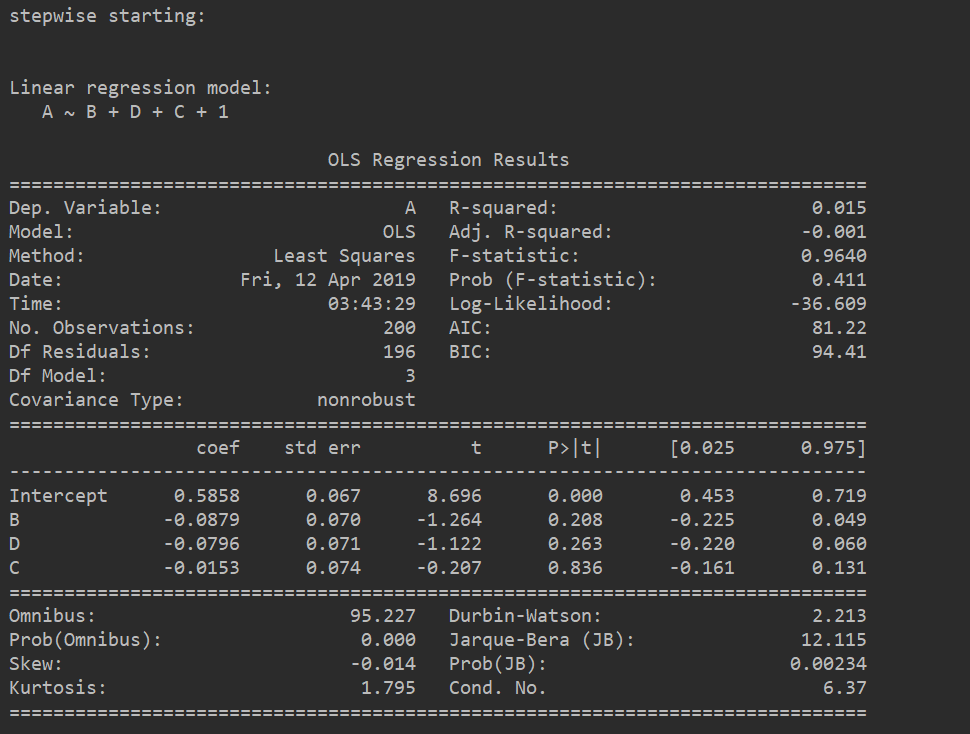注：本文所用的测试是用随机数所求，所以逐步回归的结果大部分并没有通过检验

方法是基于python3.6版本开发，里面的py包不同版本可能存在差异，使用时需要谨慎。

展开全文qq_35007162 2019-04-12 04:19:41
• 线性回归——岭回归、lasso、前向逐步回归 岭回归 lasso 前向逐步回归

线性回归——岭回归、lasso、前向逐步回归1.岭回归2.lasso3.前向逐步回归 接上一篇文章线性回归——局部加权线性回归，我们知道如何解决欠拟合，现在我们介绍一下当出现过拟合时我们怎么解决。 解决过拟合我们可以...

线性回归——岭回归、lasso、前向逐步回归

接上一篇文章线性回归——局部加权线性回归，我们知道如何解决线性回归中的欠拟合，现在我们介绍一下当出现过拟合时我们怎么解决。

首先回顾一下我们的标准线性回归中我们的回归系数 θ = ( X T X ) − 1 X T y \theta=(X^TX)^{-1}X^Ty ，其中涉及到求逆矩阵，但是实际会存在这种情况，即我们的样本非常少或者特征数太多，导致样本m小于特征数n，会导致 X T X X^TX 不可逆。从数学角度是不可逆的，也就不能直接求解，线性上我们也可以知道这会导致过拟合，因为样本太少或模型太过复杂很容易就过拟合了。
解决过拟合我们可以增加训练集（并不一定有效）、可以减少特征、也可通过正则化。
这里我们就主要介绍正则化的使用：
正则化的一般形式就是在我们的代价函数后面加入惩罚项，通过设置惩罚项让回归系数减小。一般有如下两种正则化的方式：
L1：lasso，惩罚项为 λ ∑ i = 1 m ∣ θ i ∣ \lambda\sum_{i=1}^m|\theta_i|
L2：ridge（岭回归），惩罚项为 λ ∑ i = 1 m θ i 2 \lambda\sum_{i=1}^m\theta_i^2

1.岭回归

（1）代价函数：
J ( θ ) = ∑ i = 1 m ( f ( x i ) − y i ) 2 + λ ∑ i = 1 m θ i 2 J(\theta)=\sum_{i=1}^m(f(x_i)-y_i)^2+\lambda\sum_{i=1}^m\theta_i^2
其中 f ( x i ) f(x_i) 是我们的预测值， f ( x i ) = θ T x i f(x_i)=\theta^Tx_i 。现在我们通过正规方程的解法来求解最优解，首先将我们的代价函数写矩阵的形式：
J ( θ ) = ( X θ − y ) T ( X θ − y ) + λ θ T θ J(\theta)=(X\theta-y)^T(X\theta-y)+\lambda\theta^T\theta
其中， θ = ( θ 1 θ 2 . . . θ n ) \theta=\begin{pmatrix}\theta_1\\\theta_2\\...\\\theta_n\end{pmatrix} ，现在我们将其展开：
J ( θ ) = ( θ T X − y T ) ( X θ − y ) + λ θ T θ = θ T X T X θ − θ T X y − y T X θ + y T y + λ θ T θ = θ T ( X T X + λ I ) θ − θ T X y − y T X θ + y T y J(\theta)=(\theta^TX-y^T)(X\theta-y)+\lambda\theta^T\theta\\=\theta^TX^TX\theta-\theta^TXy-y^TX\theta+y^Ty+\lambda\theta^T\theta\\=\theta^T(X^TX+\lambda I)\theta-\theta^TXy-y^TX\theta+y^Ty
θ \theta 求导:
δ J ( θ ) δ θ = ( X T X + λ I + ( X T X + λ I ) T ) θ − X T y − ( y T X ) T = 0 = ( 2 X T X + 2 λ I ) θ − 2 X T y = 0 = 2 ( X T X + λ I ) θ = 2 X T y    ⟹    θ = ( X X T + λ I ) − 1 X T y \frac{\delta J(\theta)}{\delta \theta}=(X^TX+\lambda I+(X^TX+\lambda I)^T)\theta-X^Ty-(y^TX)^T=0\\= (2X^TX+2\lambda I)\theta-2X^Ty=0\\= 2(X^TX+\lambda I)\theta=2X^Ty\\\implies \theta=(XX^T+\lambda I)^{-1}X^Ty
这里我们用到了一些常用的求导矩阵求导公式
1. δ X T A X δ X = ( A + A T ) X \frac{\delta X^TAX}{\delta X}=(A+A^T)X

2. δ A X δ X = A T \frac{\delta AX}{\delta X}=A^T

3. δ X T A δ X = A \frac{\delta X^TA}{\delta X}=A
顺便补充一下矩阵求导的知识：矩阵求导、几种重要的矩阵及常用的矩阵求导公式

（2）python代码实现：
这里用的是《机器学习实战》里面的实现：
2.1算法实现：

from numpy import *
def ridgeRegres(xMat, yMat, lam=0.2):
xTx = xMat.T*xMat
denom = xTx + eye(shape(xMat))*lam
if linalg.det(denom) == 0.0:  //判断是否可逆
print("This matrix is singular, connot do inverse")
return
ws = denom.I * (xMat.T*yMat)
return ws

def ridgeTest(xArr, yArr):
xMat = mat(xArr); yMat=mat(yArr).T
#数据标准化
yMean = mean(yMat,0)  #求均值
yMat = yMat - yMean  #这里我也不知道为什么要对y也进行中心化
xMeans = mean(xMat,0)  #求均值
xVar = var(xMat,0) #求方差
xMat = (xMat - xMeans)/xVar  #标准化
numTestPts = 30
wMat = zeros((numTestPts,shape(xMat)))
for i in range(numTestPts):
ws = ridgeRegres(xMat, yMat, exp(i-10))  #测试不同的lambda
wMat[i,:] = ws.T
return wMat

2.2绘制岭迹图：
上面我们得到了30个 λ \lambda 值对应的回归系数，现在我们绘制出回归系数随 λ \lambda 变化的曲线：

import matplotlib.pyplot as plt
import regression

ridgeWeights = regression.ridgeTest(abX,abY)
fig = plt.figure()
ax.plot(ridgeWeights)
plt.show()

结果如下：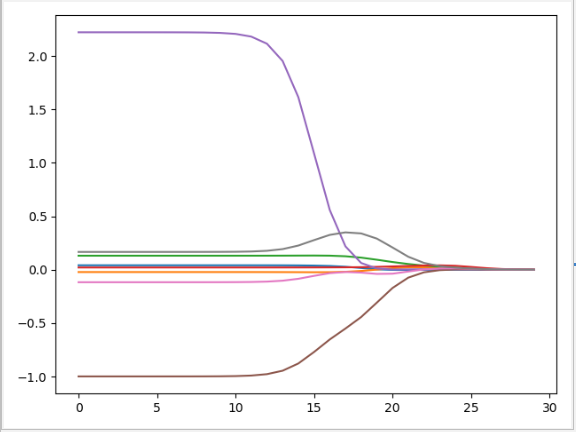可以看到当 λ \lambda 很小时，得到所有系数的原始值(与标准线性回归相同)；当 λ \lambda 很大时，得到所有都趋近0。如果要选出最优的回归系数，我们还需要进行交叉验证，选出对应误差最小的回归系数。
（3）岭回归的几何意义：
首先我们将我们上面的优化代价函数求回归系数的问题转换为如下形式：
{ J ( θ ) = ∑ i = 1 m ( f ( x i ) − y i ) 2 s . t . ∑ i = 1 m θ i 2 ≤ t \begin{cases}J(\theta)=\sum_{i=1}^m(f(x_i)-y_i)^2\\s.t. \sum_{i=1}^m\theta_i^2\leq t\end{cases}
然后我们考虑只有两个变量的情况，限制条件 θ 1 2 + θ 2 2 ≤ t \theta_1^2+\theta_2^2\leq t 就是一个圆柱，而我们的 J ( θ ) J(\theta) 就是一个抛物面，这里我们用等值线来表示，就是将这个三维的立体图映射到二维平面中，这个时候等值线与圆相切的点便是在约束条件下的最优点，因为越往外扩代价函数值就越大：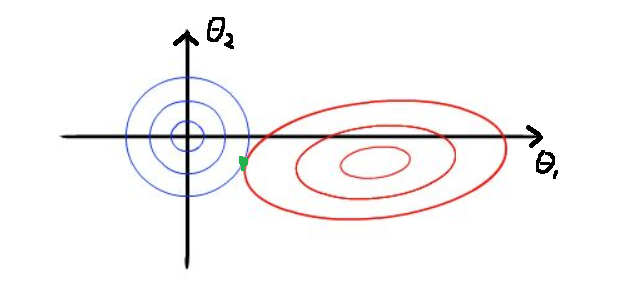2.lasso

（1）代价函数：
J ( θ ) = ∑ i = 1 m ( f ( x i ) − y i ) 2 + λ ∑ i = 1 m ∣ θ i ∣ J(\theta)=\sum_{i=1}^m(f(x_i)-y_i)^2+\lambda\sum_{i=1}^m|\theta_i|
lasso的惩罚项变成了一次： λ ∑ i = 1 m ∣ θ i ∣ \lambda\sum_{i=1}^m|\theta_i| ，使用绝对值取代了岭回归中的平方和，虽然变化不大，但是结果却大相径庭，当 λ \lambda 很小时，一些系数会随着变为0，但是岭回归却很难使得某个系数恰好缩减为0，这一点我们可以通过比较他们的几何意义了解到。
（2）lasso的几何意义：
这里我们同样以两个变量的情况进行说明，画出他们的等值线，图中lasso的约束条件 ∣ θ 1 ∣ + ∣ θ 2 ∣ ≤ t |\theta_1|+|\theta_2|\leq t 不同于岭回归，这里使用方形表示（两个变量的情况），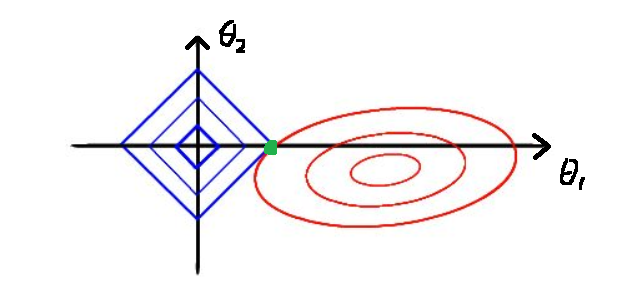方形与我们抛物面相交就是到我们的回归系数的解，相比圆，方形的顶点更容易与抛物面相交，并且其中一旦方形的顶点与抛物线（如上图中绿色的点），就会导致 θ 2 \theta_2 等于0，但是在岭回归中没有这样的点（概率很小），所以出现岭回归系数为0的概率就非常小。这也就意味着，lasso起到了很好的筛选变量的作用。

具体的代码实现就不讲了，因为可以有更简单但是效果差不多的实现方法——前向逐步回归。

3.前向逐步回归

前向逐步回归可以得到与lasso差不多的效果，但更加简单，它属于一种贪心算法，每一步都尽可能减少误差。每一步所做的决策是对某个系数增加或减少一个很小的值。
python代码实现：

from numpy import *
def regularize(xMat):#数据标准化
inMat = xMat.copy()
inMeans = mean(inMat,0)   #计算均值
inVar = var(inMat,0)      #计算方差
inMat = (inMat - inMeans)/inVar
return inMat

return ((yArr-yHatArr)**2).sum()

def stageWise(xArr,yArr,eps=0.01,numIt = 100): #numIt是我们的迭代次数，eps是我们每次迭代更新的步长
xMat = mat(xArr); yMat = mat(yArr).T
yMean = mean(yMat,0)
yMat = yMat - yMean
xMat = regularize(xMat)
m,n = shape(xMat)
returnMat = zeros((numIt,n))
ws = zeros((n,1))
wsTest = ws.copy()
wsMax = ws.copy()
for i in range(numIt):  #循环迭代numIt次
print(ws.T)
lowestError = inf
#每一次我们只对一个系数进行更新，因此我们需要循环每一个特征值，找出误差最小的回
#归系数作为下一次迭代进行更新
for j in range(n):
wsTest = ws.copy()
wsTest[j] += eps*sign
yTest = xMat*wsTest
wsMax = wsTest
ws = wsMax.copy()
returnMat[i,:]=ws.T
return returnMat

绘制结果

import matplotlib.pyplot as plt
import regression

ridgeWeights = regression.stageWise(abX,abY,0.005,1000)
fig = plt.figure()
ax.plot(ridgeWeights)
plt.show()

eps=0.005,numIt=1000的结果如下：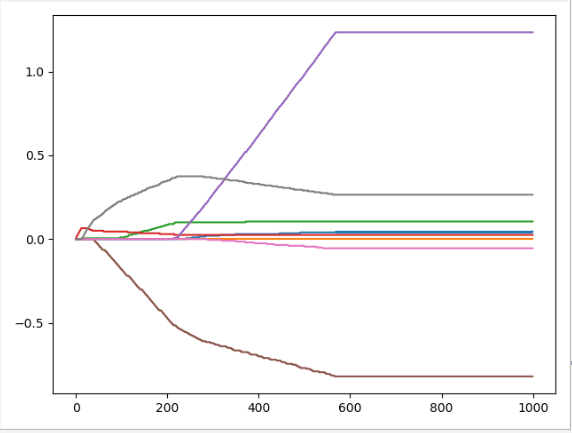展开全文CarryLvan 2019-11-12 13:06:32...